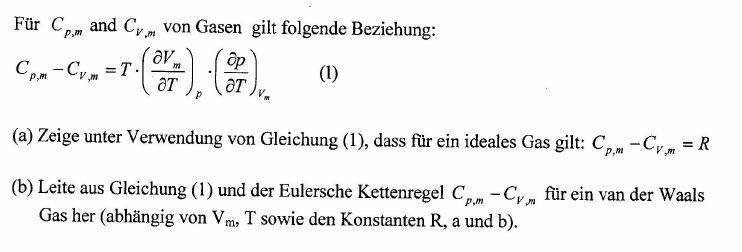# Van der Waals Gas

krootox217

## Homework Statement

Hi, I have the following task:Translated into English, that means:

" For Cpm and Cvm of gases the following relationship is true: (1)
a) Show with the relationship (1), that for an ideal gas Cpm - Cvm = R is valid
b) Deduce from equation (1) and the tripple product rule Cpm - Cvm for a Van der Waals gas (dependent on Vm, T and also R, a and b)"

I managed to solve a) but I don't know how to solve b)
Can someone help me?

## Homework Equations

Tripple product rule: https://en.wikipedia.org/wiki/Triple_product_rule
Van der Waals equation: https://de.wikipedia.org/wiki/Van-der-Waals-Gleichung

## The Attempt at a Solution

see above

Mentor

Chet

krootox217
For a) I used p*Vm=RT

then I solved the equation once for p and once for Vm, and did the partial derivatives, which yielded T*(R/p)*(R/Vm) = Cpm- Cvm = (T*R2)/(p*Vm)

and then I said p*Vm=R*T and i reduced the fraction, which yielded Cpm- Cvm = R

But I don't understand how to start in the second task, could you help me?

Mentor
I would start out by evaluating each of the partial derivatives in Eqn. 1 and see what I get. If that doesn't give me what I'm looking for, I would start thinking about ways in which the triple product rule might get me to where I want to be. Maybe you're going to have to "play with the math" at little.

Chet

krootox217
Did I understand you correctly that I should do the same as in a), but this time not with the ideal gas equation, but with the van der waals equation?

Mentor
Did I understand you correctly that I should do the same as in a), but this time not with the ideal gas equation, but with the van der waals equation?
Sure. The problem statement indicates that the final answer should include all the parameters except for P. To me, this means that the first partial derivative in Eqn. 1 might be troublesome. See how it all plays out. Start by evaluating the two partial derivatives in equation 1 based on the van der waals equation.

•krootox217
krootox217
Ok, thanks a lot! :)